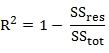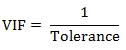# SQL Server VIF function

VIF

Updated: 27 February 2015

Use the table-valued function VIF to calculate the R2, tolerance, and the variance inflation factor for a set of independent variables.
VIF performs a linear regression for each independent variable against all the other independent variables. For each of these regression analyses it calculates R2 as:where SSres is the residual sum of squares and SStot is the total sum of squares.
The tolerance is derived directly from R2.The variance inflation factor is calculated from the tolerance.

Syntax

SELECT * FROM [wct].[VIF](
<@Matrix_RangeQuery, nvarchar(max),>)
@Matrix_RangeQuery
the SELECT statement, as a string, which, when executed, creates the resultant table of x-values used in the calculation.

Return Types
RETURNS TABLE (
[ColNum] [int] NULL,
[Rsquared] [float] NULL,
[VIF] [float] NULL,
[Tolerance] [float] NULL
)

Table Description
ColNum
The column number from the resultant table returned by @Matrix_RangeQuery.
Rsquared
The R2 value.
VIF
The variance inflation factor.
Tolerance
The tolerance.

Remarks
·         @Matrix_RangeQuery must return at least 2 columns or an error will be returned.
·         If Rsquared = 1 then Tolerance is zero and VIF is NULL.
·         If there is only one column of data, no rows will be returned.

Examples
In this example we evaluate 3 independent variables: age, height, and weight.
SELECT
*
INTO
#t
FROM (VALUES
(22,76,197.4)
,(27,68.9,189.3)
,(28,67.7,215.1)
,(20,72.3,173.6)
,(29,71.2,171)
,(23,70.3,184.6)
,(20,73.4,178.7)
,(23,64.6,217)
,(23,68.2,204)
,(24,78.9,249.9)
,(25,76.3,209.3)
,(22,78.8,209.7)
,(26,61.4,137.3)
,(24,73.4,186.2)
,(28,76.5,220.4)
,(20,75.9,171.8)
,(25,77.1,239.3)
,(25,67.3,169.9)
,(28,71.8,184.3)
,(21,77.6,180.3)
)n(Age,Height,Weight)

This produces the following result.
ColNum               Rsquared                    VIF              Tolerance
----------- ---------------------- ---------------------- ----------------------
1      0.166469615505746        1.1997163134092      0.833530384494254
2      0.349782414258577       1.53794671495963      0.650217585741423
3      0.296159110711204       1.42077565429661      0.703840889288796

While we put our data into a temporary table (#t) and executed the table-valued function by referencing #t, we could have just as easily put the SQL inside the call to the function as the following SQL demonstrates.
SELECT
*
FROM wct.VIF('
SELECT
*
FROM (VALUES
(22,76,197.4)
,(27,68.9,189.3)
,(28,67.7,215.1)
,(20,72.3,173.6)
,(29,71.2,171)
,(23,70.3,184.6)
,(20,73.4,178.7)
,(23,64.6,217)
,(23,68.2,204)
,(24,78.9,249.9)
,(25,76.3,209.3)
,(22,78.8,209.7)
,(26,61.4,137.3)
,(24,73.4,186.2)
,(28,76.5,220.4)
,(20,75.9,171.8)
,(25,77.1,239.3)
,(25,67.3,169.9)
,(28,71.8,184.3)
,(21,77.6,180.3)
)n(Age,Height,Weight)'
)

Since the table-valued function uses dynamic SQL, however, it will not produce the desired result if it references a derived table or a common table expression outside the scope of the dynamic SQL. For example, the following SQL will not work.
WITH mycte AS (
SELECT
*
FROM (VALUES
(22,76,197.4)
,(27,68.9,189.3)
,(28,67.7,215.1)
,(20,72.3,173.6)
,(29,71.2,171)
,(23,70.3,184.6)
,(20,73.4,178.7)
,(23,64.6,217)
,(23,68.2,204)
,(24,78.9,249.9)
,(25,76.3,209.3)
,(22,78.8,209.7)
,(26,61.4,137.3)
,(24,73.4,186.2)
,(28,76.5,220.4)
,(20,75.9,171.8)
,(25,77.1,239.3)
,(25,67.3,169.9)
,(28,71.8,184.3)
,(21,77.6,180.3)
)n(Age,Height,Weight)
)
SELECT
*
FROM
wct.VIF('SELECT * FROM mycte')

This produces the following message.
Exception:
[Product version 1.13 Build: 0219.573]
An error occurred in Function Package while attempting to access database data:
Invalid object name 'mycte'. (msg:208 state:1 ln:0)

Error attempting to read database data. Ref-Code: 1-g

RangeQuery:
SELECT * FROM mycte
XLeratorDB_stats2008.Core.Exceptions+Function_RangeQuery_Exception:
at a.a(String A_0, String A_1, String A_2)
at a.v.d()
at a.v.d(String A_0, String A_1, String A_2, Boolean A_3)
at XLeratorDB_stats2008.UserDefinedFunctions.VIF(SqlString Matrix_RangeQuery)

In this example we generate a random variable (x1) from the normal distribution with a mean of 0 and standard deviation of 1, another random variable (x2) from the normal distribution a mean of 2 and a standard deviation
of 4. The x3 variable is calculated as x2 plus a uniform random variable between 0 and 1.
SELECT
x1
,x2
,x2 + wct.RAND() as x3
INTO
#t1
FROM (
SELECT
X as X1
,wct.RANDNORM(2,4) as X2
FROM
wct.RANDSNORMAL(1000)
)n

SELECT
*
FROM
wct.VIF('SELECT x1,x2,x3 FROM #t1')

This produces the following result (your result will be different).
ColNum               Rsquared                    VIF              Tolerance
----------- ---------------------- ---------------------- ----------------------
1   0.000933473047366573       1.00093434523346      0.999066526952633
2      0.994621815206927       185.936340693973    0.00537818479307317
3      0.994621666804285       185.931210211501    0.00537833319571512

As expected the R2 between x2 and x3 is very close to and the VIF for the 2 columns is very high.

In this example, we generate 3 independent random variables and a fourth variable which is calculated as x1 + 2*x2 - 3*x3. In other words, co-linearity arises not between any two columns but among all the columns.
SELECT
x1
,x2
,x3
,x1 + 2*x2 - 3*x3 as x4
INTO
#t2
FROM (
SELECT
X as X1
,wct.RANDNORM(2,4) as X2
,wct.RANDNORM(5,10) as X3
FROM
wct.RANDSNORMAL(1000)
)n

This produces the following result (your result will be different).
ColNum               Rsquared                    VIF              Tolerance
----------- ---------------------- ---------------------- ----------------------
1                      1                   NULL                      0
2                      1                   NULL                      0
3                      1                   NULL                      0
4                      1                   NULL                      0

Eliminating any of the columns will eliminate the co-linearity.
SELECT
*
FROM
wct.VIF('SELECT x1,x2,x4 FROM #t2')

This produces the following result (your result will be different).
ColNum               Rsquared                    VIF              Tolerance
----------- ---------------------- ---------------------- ----------------------
1   8.47196531994499E-05       1.00008472683123      0.999915280346801
2     0.0746046878401549       1.08061926277326      0.925395312159845
3     0.0746307067340327       1.08064964687842      0.925369293265967

### SupportCopyright 2008-2023 Westclintech LLC         Privacy Policy        Terms of Service